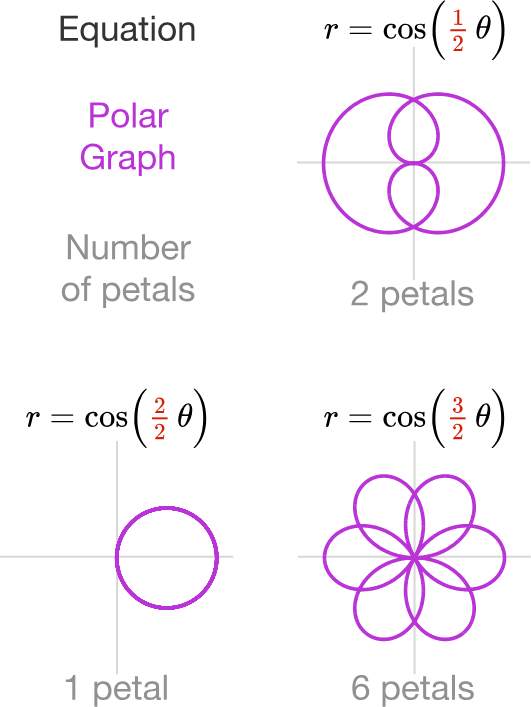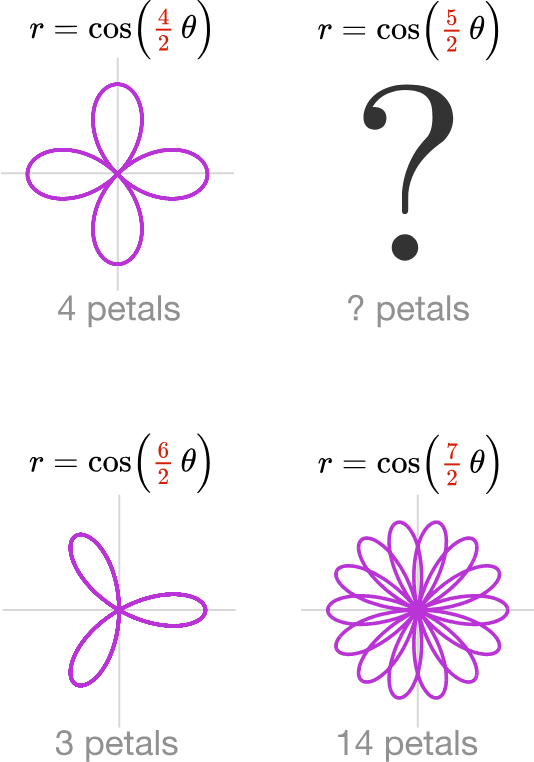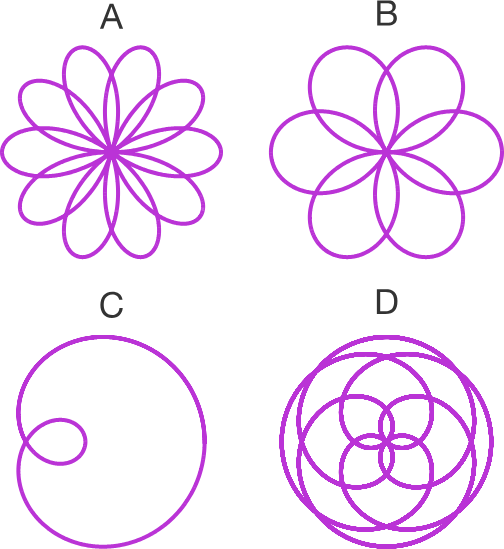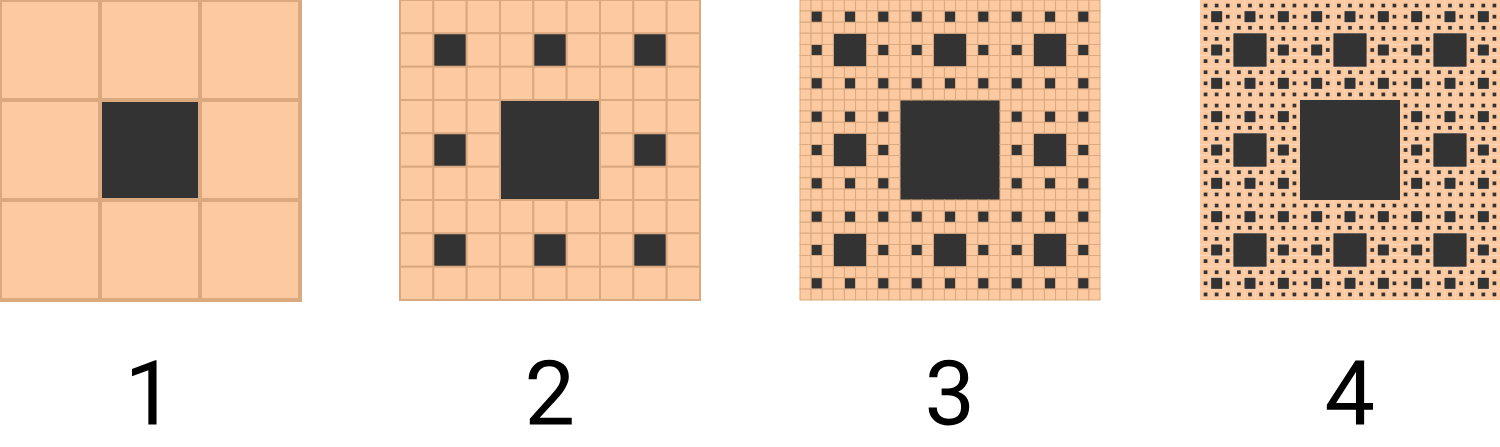### Complex Numbers

Now that you've gotten a taste for algebraic intuition, here are three reasons to dive in and complete everything:

1. To fully master Algebra. (Note: this is not an introductory Algebra sequence, it is intended for people looking to master Algebra at a challenging and complex level.)

2. To understand Euler's identity: $e^{i \pi} + 1 = 0.$

3. To explore the applications of complex algebra to art and animation. (To learn how to create CGI and fractals.)

This next section will introduce each of the three motivations above in more detail and give you a preview of what you can learn here.

Don't worry if you get a few or even all of these questions wrong; they are questions that demonstrate that there is a lot of very challenging math in this topic area, Complex Algebra.

# Why Complete This Course?

One of the biggest advantages in how this course approaches Algebra is that we’ll be using complex numbers and the complex plane throughout the whole course in order to demonstrate why complex numbers make a lot of Algebra more natural, not less.

That said, mastering complex numbers will be a challenge!

What is $\left(\sqrt{-4}\right)\left(\sqrt{-9}\right)?$

Note: The complex number $i$ is defined as $i = \sqrt{-1}.$ For positive $a,$ $\sqrt{-a} = i \sqrt{a}.$

# Why Complete This Course?

Motivation $1:$

To fully master Algebra, so as to be able to use it fluently and creatively, not just by applying memorized procedures.

For example, even if you've never seen polar equations like the ones below before, you can still solve this puzzle just by thinking about how the images and equations relate to each other. The graphs below are all called "roses":Which of the following is the missing rose?# Why Complete This Course?

Motivation $2:$

To understand how complex numbers fit into mathematics and what Euler's mind-blowing identity $e^{i \pi} + 1 = 0$ really means.

True or False?

$e^{\color{#D61F06}{-i \pi}} + 1 = 0$

Note: Careful! Note the negative sign in the exponent on the left hand side of the equation.

# Why Complete This Course?

Motivation $3 :$

To extend the mathematics in this course to applications creating animated graphics and mathematical art in the form of fractals.Suppose a copper plate on a circuit board is made by removing squares from a square of copper so that it looks like the $4^\text{th}$ stage of the Sierpinski's carpet fractal shown above. At the fourth stage of this pattern, what fraction of the original copper square is still remaining?

# Why Complete This Course?

Consider the following "proof" that $1 = -1.$ What is the first step that is incorrect?

Step $\textbf 1:$ $\frac{-1}{1} = \frac{1}{-1}.$

Step $\textbf 2:$ Take the square root of both sides to obtain $\sqrt{\frac{-1}{1}} = \sqrt{\frac{1}{-1}}.$

Step $\textbf 3:$ Then, $\frac{\sqrt{-1}}{\sqrt{1}} = \frac{\sqrt{1}}{\sqrt{-1}}.$

Step $\textbf 4:$ So, $\frac{i}{1} = \frac{1}{i}.$

Step $\textbf 5:$ Multiply each side by $i$ to obtain $\frac{i^2}{1} = \frac{i}{i}.$

Step $\textbf 6:$ Simplify each fraction: $\frac{i^2}{1} = i^2$ and $\frac{i}{i} = 1.$ Then, since $i^2 = -1,$ we have the final result:
$\large -1 = 1.$

Note: The complex number $i$ is defined as $i = \sqrt{-1}.$

Overall, the questions in this course will require more thought and creativity than most problems you’d see in Algebra textbooks. Expect some of them to take you a bit longer, and expect to get many wrong--this is a hard field of mathematics to master!

×